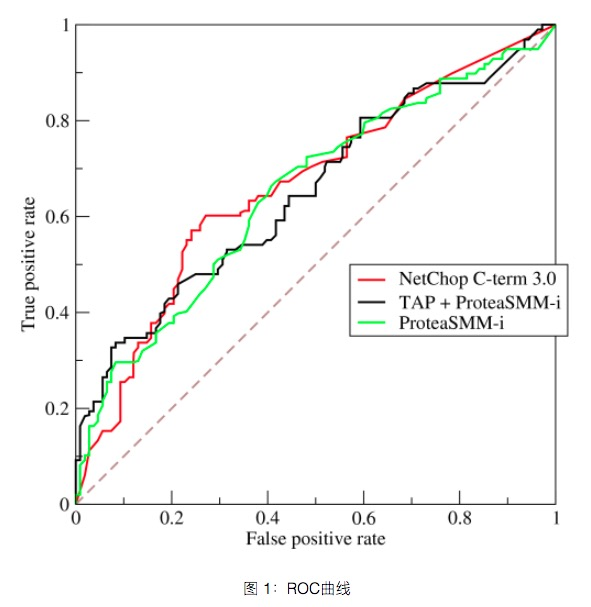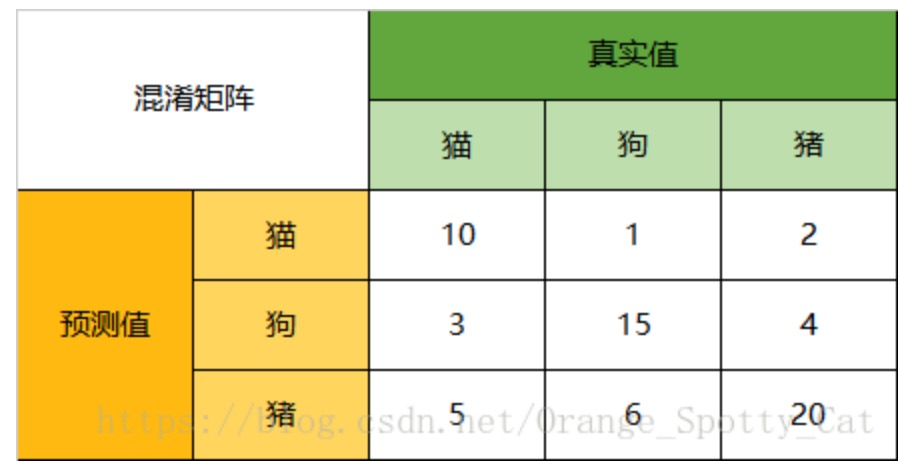# 上来先抛出一堆词，炸死你

• Recall
• Precision
• TPR
• FPR
• 准确率 （Accuracy）
• 混淆矩阵 （Confusion Matrix）
• 精确率（Precision）
• 召回率（Recall）
• 平均正确率（AP）
• mAP（mean Average Precision）
• ROC
• AUC

# 一堆概念来袭

• 你关注负类么？ 因为二分类的时候，你可能不是光关注正类，可能也非常关注负类，这样，就要“平等”对待它们。 但是对目标检测的情形，你更关心的正类，你是带着“偏见”的，体现在指标上，也是有区别的。

## TP、FP、FN、TN

• TP 你预测的正例里面，那些真的正例
• FP 你预测的正例里面，那些假的正例（人家其实是真负例）
• FN 你预测的负例里面，那些假的负例（人家其实是真正例）
• TN 你预测的负例里面，那些真的负例

## 准确率 - accuracy

（ 你预测对的正例+你预测对的负例 ）/ 样本总数

$\begin{equation}\label{equ:accuracy} \mbox{accuracy} = \frac{TP+TN}{TP+TN+FP+FN} = \frac{TP+TN }{\mbox{all data}} \end{equation}$

## 精确率 - precision（查准率）

$\begin{equation}\label{equ:precision} \mbox{precision} = \frac{TP}{TP+FP} = \frac{TP}{\mbox{预测为positive的样本}} \end{equation}$

## 召回率 - recall（查全率）

$\begin{equation}\label{equ:recall} \mbox{recall} = \frac{TP}{TP+FN} = \frac{TP}{\mbox{真实为positive的样本}} \end{equation}$

## ROC曲线• 纵坐标True Positive Rate（TPR），其实就是正类的召回率：$recall_+$。
$\begin{equation}\label{equ:tpr} \begin{split} \mbox{TPR} &= \frac{TP}{TP + FN} \\ &= \mbox{recall_{positive}} \end{split} \end{equation}$
• 横坐标False Positive Rate（FPR），其实就是1-负类的召回率：1-$recall_{-}$。
$\begin{equation}\label{equ:fpr} \begin{split} \mbox{FPR} &= \frac{FP}{FP + TN} = \frac{FP + TN -TN}{FP + TN} \\ &= 1 - \frac{TN}{FP + TN} \\ &= 1 - \mbox{recall_{negative}} \end{split} \end{equation}$

## 混淆矩阵• TP 你预测的正例里面，那些真的正例
• FP 你预测的正例里面，那些假的正例（人家其实是真负例）
• FN 你预测的负例里面，那些假的负例（人家其实是真正例）
• TN 你预测的负例里面，那些真的负例TP这里的含义没变，就是“标注为正例实际上也是正例”，这里，只要没分对，就是负例，比如猫那行，10个猫被分对了，1只被错误分成了狗，2只被错误分成了猪）。

• 精确率precision = 10/（10+1+2）
• 召回率recall = 10/（10+3+5）

F1根据精确率和召回率可以算出，不赘述了。

## PR曲线

PR曲线很容易和ROC曲线，她们长的很像，也有类似的功效，但是他们确实不同的。

PR曲线的坐标不在是TPR和FPR了，而是改成了精确率precision和召回率recall。## 总结一下

• 2分类，还是多分类：2分类好理解recall、precision、F1
• 2分类评价再进一步，高出2分类混淆矩阵、ROC曲线、AUC面积
• 多分类，那就把这些概念扩展起来，绕个弯，也很容易搞定
• 只关心正例，那就引出PR曲线和AP面积、多分类mAP了